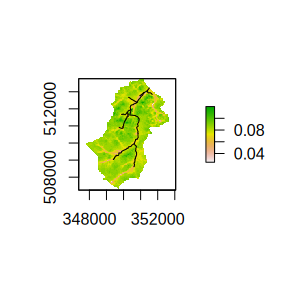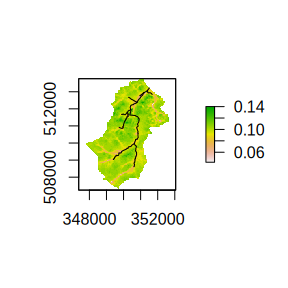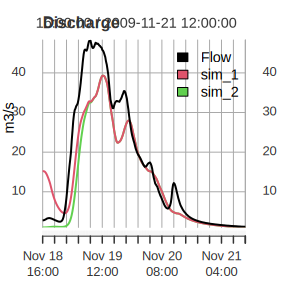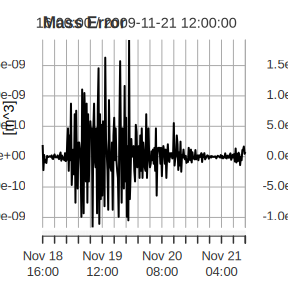# Using Dynamic TOPMODEL

The purpose of this vignette is to provide an outline of the steps needed to perform a Dynamic TOPMODEL simulation and introduce the formats of the data input and returned.

The data used in this example comes from Swindale and is contained within the package and can be loaded with

library(dynatop)
data("Swindale")

which returns a variable Swindale with the following components:

names(Swindale)
#>  "model" "obs"

For better comparison with a likely analysis we separate these into a model and observed data variables

swindale_model <- Swindale$model swindale_obs <- Swindale$obs

# The model structure

A dynamic TOPMODEL is described in a list object. The list has the following elements

names(swindale_model)
#>   "options"        "map"            "channel"        "hillslope"
#>   "flow_direction" "gauge"          "point_inflow"   "diffuse_inflow"
#>   "precip_input"   "pet_input"

which are described in associated vignette. The dynatopGIS package can be used for constructing models.

# Setting map locations

While not required for simulations if the locations of the files containing the locations of the HRUs are provided the states can be visualised within dynatop.

The locations of the files are set in the map element of the model. For this example the maps are located within the extdata directory of the package and can be set using commands

swindale_model$map$hillslope <- system.file("extdata","Swindale.tif",package="dynatop",mustWork=TRUE)
swindale_model$map$channel <- system.file("extdata","channel.shp",package="dynatop",mustWork=TRUE)
swindale_model$map$channel_id <- system.file("extdata","channel_id.tif",package="dynatop",mustWork=TRUE)

# Preparing input data

The input to the model is expected to take the form of an xts object with constant time step whose column names are found in the ‘precip’ and ‘pet’ columns of the HRU tables in the model. Helpful functions for creating and manipulating xts objects can be found here, see also the resample_xts function in this package.

The discharge, precipitation and potential evapotranspiration (PET) inputs for Swindale are contained with swindale_obs on a 15 minute time step.

head(swindale_obs)
#> Warning: timezone of object (GMT) is different than current timezone ().
#>                     Flow Rainfall          PET
#> 2009-11-18 16:00:00 2.78    4e-04 8.878467e-06
#> 2009-11-18 16:15:00 2.80    2e-04 4.762229e-06
#> 2009-11-18 16:30:00 2.85    4e-04 8.585708e-07
#> 2009-11-18 16:45:00 2.94    2e-04 0.000000e+00
#> 2009-11-18 17:00:00 3.02    2e-04 0.000000e+00
#> 2009-11-18 17:15:00 3.15    2e-04 0.000000e+00

Note the discharge is in m$$^{3}$$/s while the precipitation and PET are in m accumulated over the time step.

To use the data with the model we need to set the names of the time series inputs within the model. In this case this is already done as can be seen by inspecting the precip_input and pet_input tables

head(swindale_model$precip_input) #> name id frc #> 1 Rainfall 2 1 #> 2 Rainfall 17 1 #> 3 Rainfall 11 1 #> 4 Rainfall 7 1 #> 5 Rainfall 6 1 #> 6 Rainfall 12 1 head(swindale_model$pet_input)
#>   name id frc
#> 1  PET  2   1
#> 2  PET 17   1
#> 3  PET 11   1
#> 4  PET  7   1
#> 5  PET  6   1
#> 6  PET 12   1

# Altering parameters

The parameter values are stored within the table describing the hillslope and channel HRUs. Which parameters are present depends upon the options selected for the transmissivity and channel solution. Details can be found in the Hillslope and Channel Vignettes.

Altering parameter values requires changing their values in the HRU tables. For this catchment all HRU have the same parameter values. For this simulation we change the parameter vectors to be more representative of the catchment

swindale_model$hillslope$r_sfmax <- Inf
swindale_model$hillslope$m <- 0.0063
swindale_model$hillslope$ln_t0 <- 7.46
swindale_model$hillslope$s_rz0 <- 0.98
swindale_model$hillslope$s_rzmax <- 0.1
swindale_model$hillslope$t_d <- 8*60*60
swindale_model$hillslope$c_sf <- 0.4

swindale_model$channel$v_ch <- 0.8

# Creating the dynatop Object

Simulations are performed by embedding the model and the observed data into a dynatop object. First the object is created using the model in list form

ctch_mdl <- dynatop$new(swindale_model) This step performs some basis checks on the model for consistency. The data can then be added ctch_mdl$add_data(swindale_obs)

# Running dynamic TOPMODEL

The model currently consists of two types of HRU; hillslope and channel. These can be run individually with the sim_hillslope and sim_channel methods or sequentially with the sim method. The individual methods check that suitable input data is available, but not how it was generated.

The initial states of the simulations can be specified in the model object. If, as in the case of this example, the states are not specified then any attempt to perform a simulation will fail.

ctch_mdl$sim() #> Error in self$sim_hillslope(keep_states, sub_step, tol, max_it): Model states are either not initialised or have non-finite values

The states need to be initialised using the initialise method which requires an initial recharge rate. In the following we initialise the states and plot the initial saturated zone storage deficit, using the chaining of commands.

ctch_mdl$initialise()$plot_state("s_sz")The simulation can now be performed and the flow at the gauge extracted with

sim1 <- ctch_mdl$sim()$get_gauge_flow()
#> Warning in private$sim_hs(keep_states, sub_step, tol, max_it, ftol): Courant number for surface zone is over 0.7 #> Suggest maximum sub step is: 63.11seconds #> Warning in private$sim_hs(keep_states, sub_step, tol, max_it, ftol): Courant number for saturated zone is over 0.7
#> Suggest maximum sub step is: 0seconds

Note that the states of the system are now those at the end of the simulation for example:

ctch_mdl$plot_state("s_sz")Rerunning the simulation with the new initial states will of course produce different results. Output for the above examples can be plotted against observed discharge for comparison as follows: sim2 <- ctch_mdl$sim()$get_gauge_flow() #> Warning in private$sim_hs(keep_states, sub_step, tol, max_it, ftol): Courant number for surface zone is over 0.7
#> Suggest maximum sub step is: 63.11seconds
#> Warning in private$sim_hs(keep_states, sub_step, tol, max_it, ftol): Courant number for saturated zone is over 0.7 #> Suggest maximum sub step is: 0seconds out <- merge( merge(swindale_obs,sim1),sim2) names(out) <- c(names(swindale_obs),'sim_1','sim_2') plot(out[,c('Flow','sim_1','sim_2')], main="Discharge",ylab="m3/s",legend.loc="topright")# Mass balance It is possible to output the mass balance check for each time step of the simulation using the get_mass_errors method. The returned matrix gives the volumes in the states at the start and end of the time step along with the other fluxes as volumes. This can easily be used to plot the errors as shown below. mb <- ctch_mdl$get_mass_errors()
plot( mb[,6] , main="Mass Error", ylab="[m^3]")